Question

# PRINTER VERSION BACK ESOURCES Chapter 21, Problem 07 Your answer is partially correct. Try again. Problem OR Problem...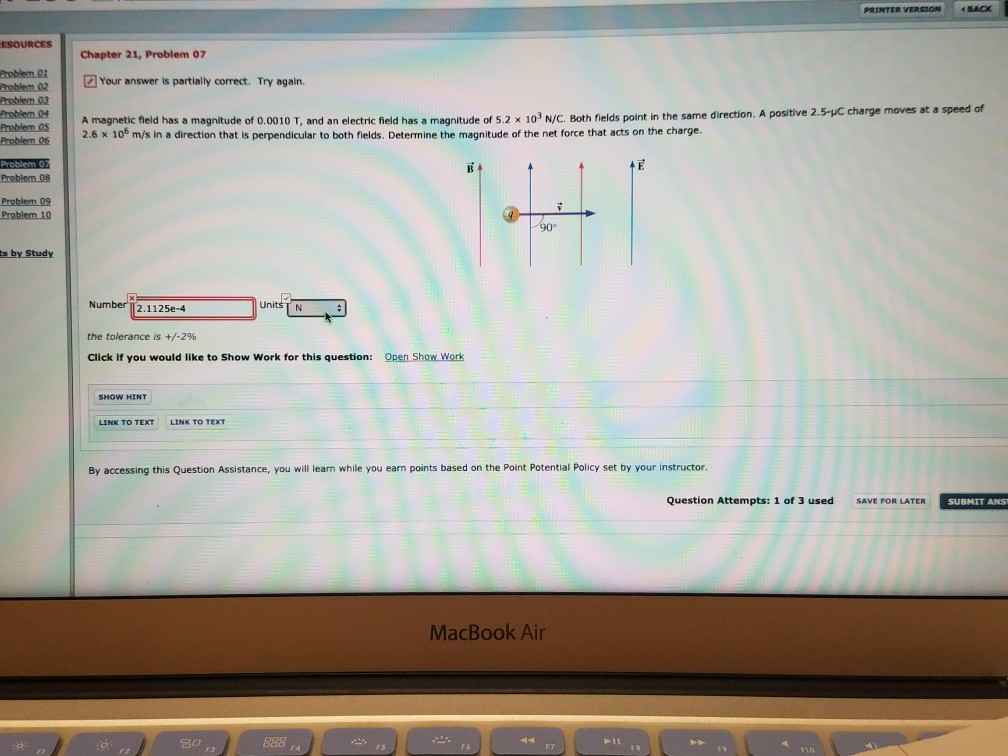PRINTER VERSION BACK ESOURCES Chapter 21, Problem 07 Your answer is partially correct. Try again. Problem OR Problem 02 Problem 03 Problem 04 Problem 05 Problem 06 A magnetic field has a magnitude of 0.0010 T, and an electric field has a magnitude of 5.2 x 10' N/C. Both fields point in the same direction. A positive 2.5-C charge moves at a speed of 2.6 X 10 m/s in a direction that is perpendicular to both fields. Determine the magnitude of the net force that acts on the charge. Problem O Problem O Problem 09 Problem 10 ts by Study Number 2.1125e-4 Units TN the tolerance is +/-2% Click if you would like to Show Work for this question: Open Show Work SHOW HINT LINK TO TEXT LINK TO TEXT By accessing this Question Assistance, you will learn while you earn points based on the Point Potential Policy set by your instructor. Question Attempts: 1 of 3 used SAVE FOR LATER SUBMIT ANSI MacBook Air 30 - no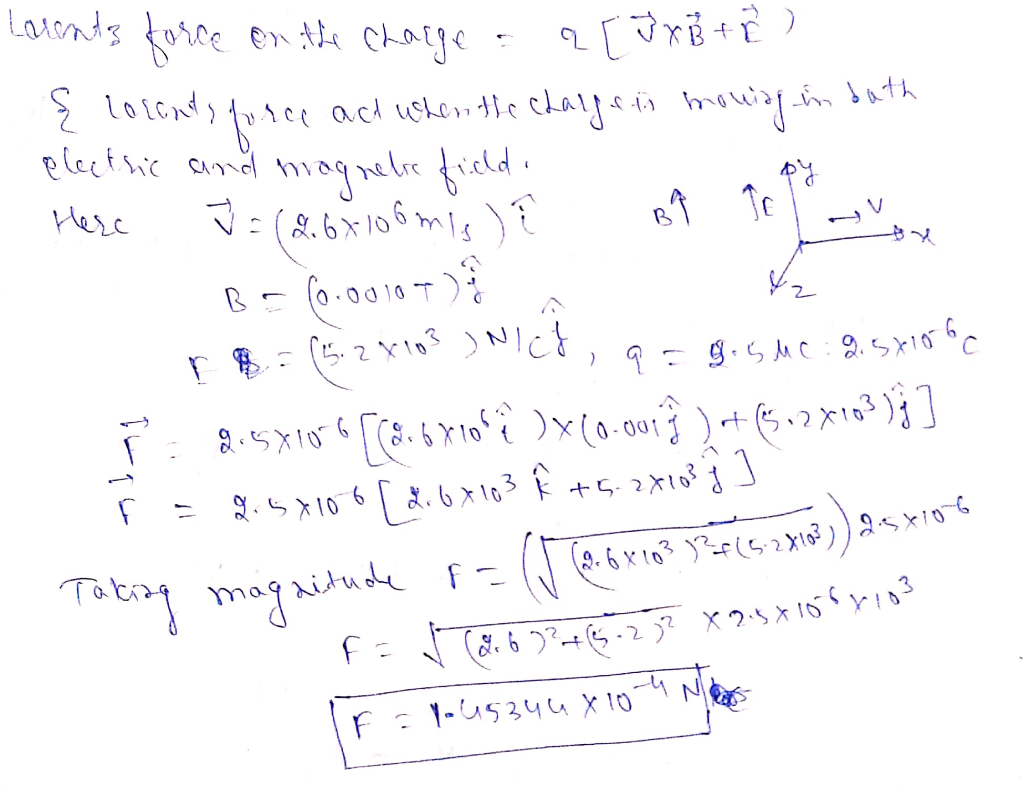#### Earn Coins

Coins can be redeemed for fabulous gifts.

Similar Homework Help Questions
• ### PRINTER VERSION BACK ESOURCES Chapter 21, Problem 07 Your answer is partially correct. Try again. Problem...PRINTER VERSION BACK ESOURCES Chapter 21, Problem 07 Your answer is partially correct. Try again. Problem OR Problem 02 Problem 03 Problem 04 Problem 05 Problem 06 A magnetic field has a magnitude of 0.0010 T, and an electric field has a magnitude of 5.2 x 10' N/C. Both fields point in the same direction. A positive 2.5-C charge moves at a speed of 2.6 X 10 m/s in a direction that is perpendicular to both fields. Determine the magnitude...

• ### Chapter 21, Problem 07 Your answer is partially correct. Try again. A magnetic field has a...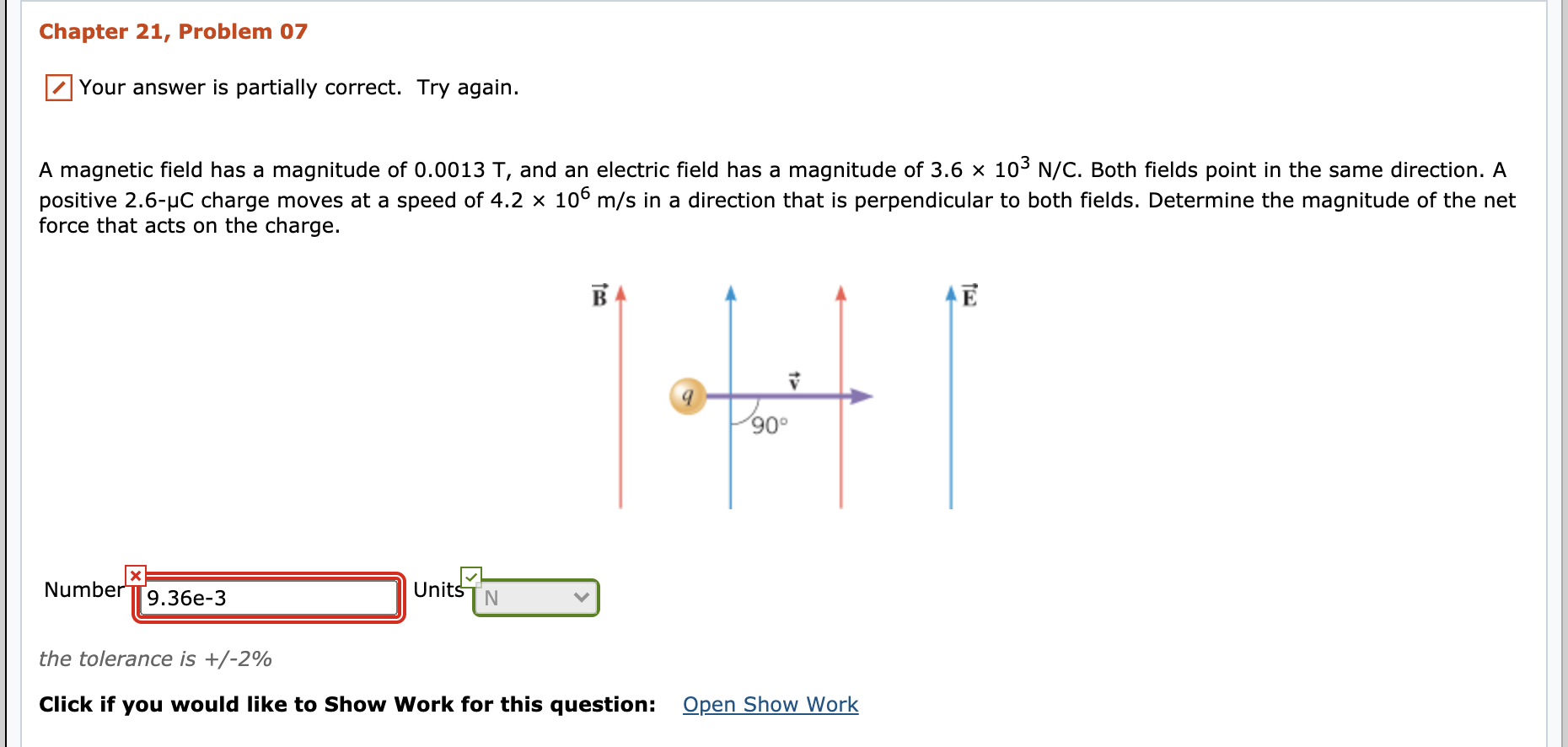Chapter 21, Problem 07 Your answer is partially correct. Try again. A magnetic field has a magnitude of 0.0013 T, and an electric field has a magnitude of 3.6 × 103 N/C. Both fields point in the same direction. A positive 2.6-4C charge moves at a speed of 4.2 x 106 m/s in a direction that is perpendicular to both fields. Determine the magnitude of the net force that acts on the charge. B Ē 9 ✓ 90° X Number...

• ### Chapter 21, Problem 07 your answer is partially correct. Try again. A magnetic field has a...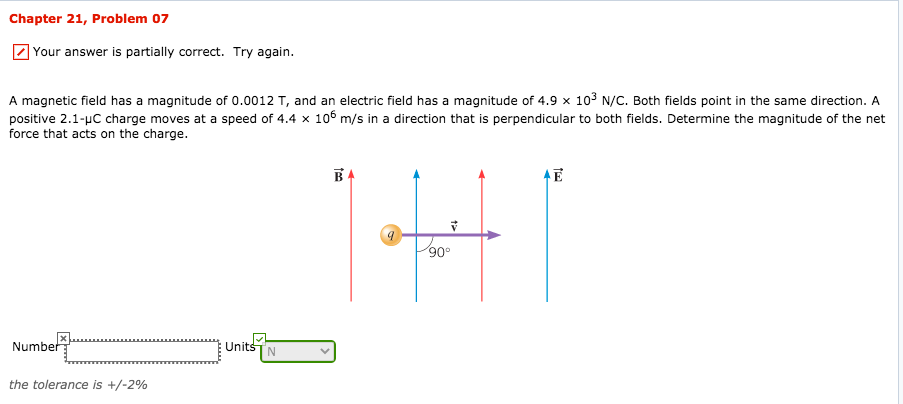Chapter 21, Problem 07 your answer is partially correct. Try again. A magnetic field has a magnitude of 0.0012 T, and an electric field has a magnitude of 4.9 x 103 N/C. Both fields point in the same direction. A positive 2.1- C charge moves at a speed of 4.4 x 106 m/s in a direction that is perpendicular to both fields. Determine the magnitude of the net force that acts on the charge. BA Ē 9 90° Number Units...

• ### nment FULL SCREEN PRINTER VERSION 4 BACK N Chapter 22, Problem 087 Your answer is partially...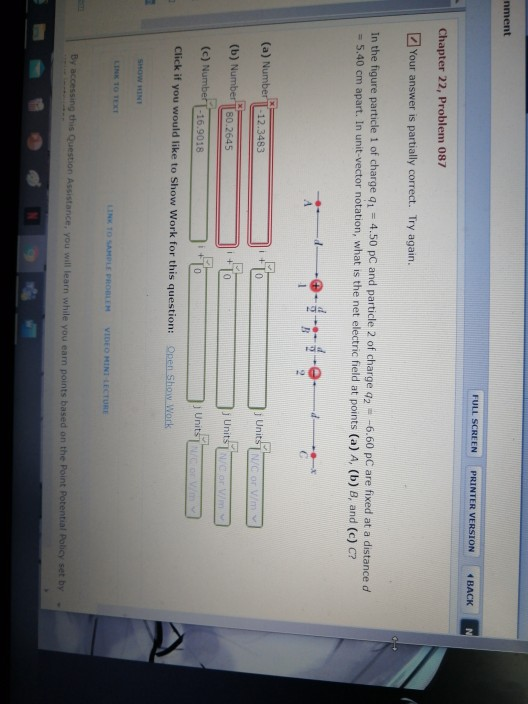nment FULL SCREEN PRINTER VERSION 4 BACK N Chapter 22, Problem 087 Your answer is partially correct. Try again. In the figure particle 1 of charge 91 = 4.50 PC and particle 2 of charge 42 - -6.60 PC are fixed at a distance d = 5.40 cm apart. In unit-vector notation, what is the net electric field at points (a) A. (b) e, and (c) C? ---- d A 1 10 (a) Number -12.3483 0 Unit NC or my...

• ### CALCULATOR FULL SCREEN PRINTER VERSION ESOURCES BACK NEXT Do It! Review 9-02b 9-09 x Your answer...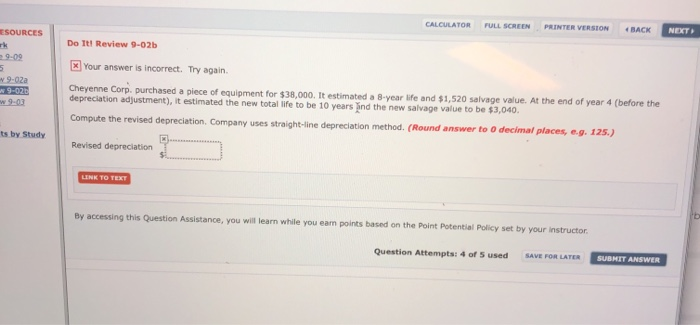CALCULATOR FULL SCREEN PRINTER VERSION ESOURCES BACK NEXT Do It! Review 9-02b 9-09 x Your answer is incorrect. Try again v9-02a w 9-026 Cheyenne Corp. purchased a piece of equipment for \$38,000. It estimated a year life and \$1,520 salvage value. At the end of year 4 (before the depreciation adjustment), it estimated the new total life to be 10 years and the new salvage value to be \$3,040. Compute the revised depreciation Company uses straight-line depreciation method. (Round answer...

• ### SCREEN PRINTER VERSION BACK Chapter 21, Problem 042 Your answer is partially correct. Try again. In...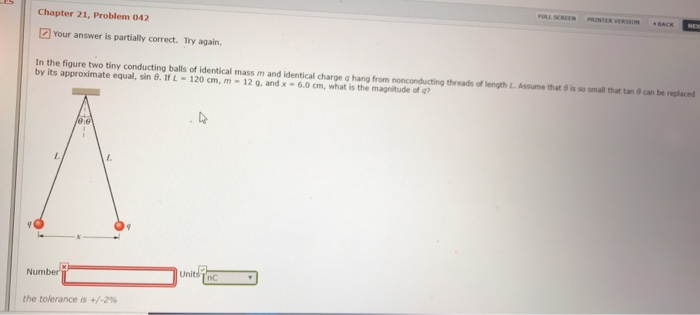SCREEN PRINTER VERSION BACK Chapter 21, Problem 042 Your answer is partially correct. Try again. In the figure two tiny conducting balls of identical mass m and identical charge a hang from no conducting threads of 1 by its approximate equal, sin θ. tfL-120 cm, m-12 g, and x-6.0cm, what h the magnitude of gth L Ass me that。S so sm a at ta e can be replaced Number nC : the tolerance is +/-2%

• ### Chapter 19, Problem 13  Your answer is partially correct. Try again. wo point charges, +2.10...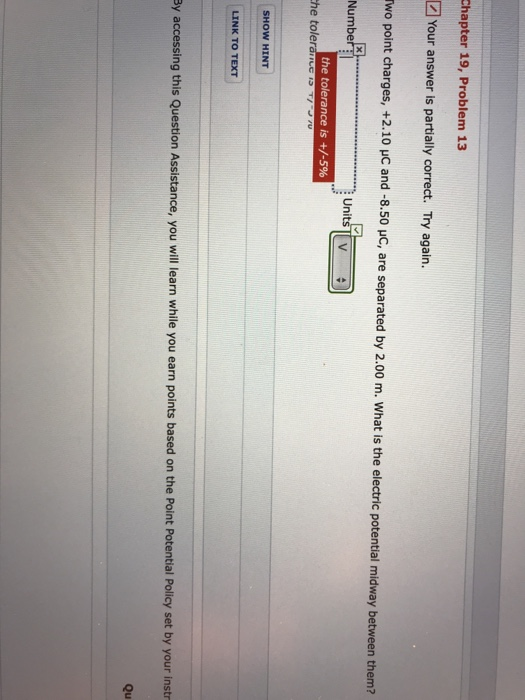Chapter 19, Problem 13  Your answer is partially correct. Try again. wo point charges, +2.10 uC and -8.50 uC, are separated by 2.00 m. What is the electric potential midway between them? Numbe UnitsV the tolerance is +/-596 the tole SHOW HINT LINK TO TEXT By accessing this Question Assistance, you will learn while you earn points based on the Point Potential Policy set by your instr Qu Charges of q and +2g are fixed in place, with a...

• ### Chapter 18, Problem 19 Your answer is partially correct. Try again. provides some pertinent background for...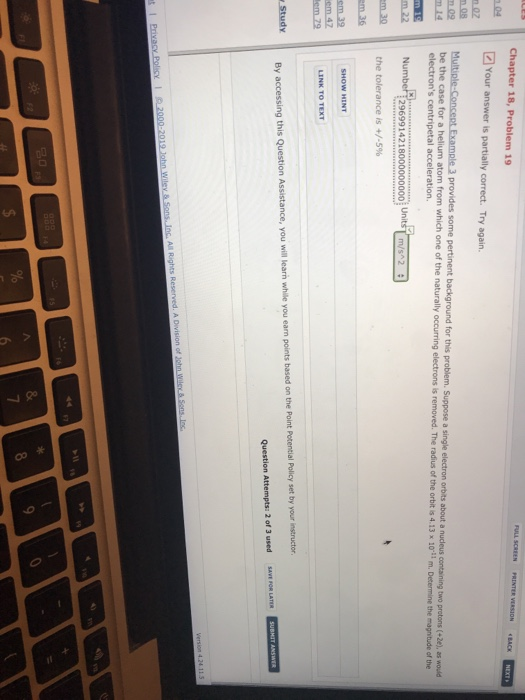Chapter 18, Problem 19 Your answer is partially correct. Try again. provides some pertinent background for this problem. Suppose a single electron orbits about a nucleus containing two protons (+2e), as would be the case for a helium atom from which one of the naturally ng electrons is removed. The radius of the orbit is 4.13 x 10-11 m. Determine the magnitude of the electron's centripetal acceleration. Number2969914218000000000 the tolerance is +/-5% UnitsT m/s 2 LINK TO TEXT By accessing...

• ### Chapter 19, Problem 13 Your answer is partially correct. Try again. Two point charges, +3.10 C...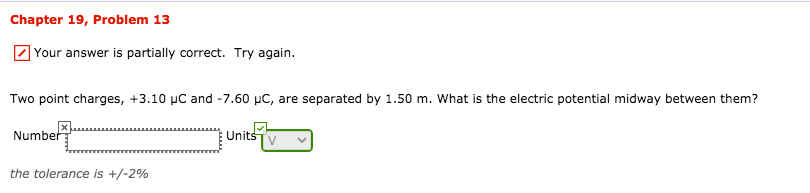Chapter 19, Problem 13 Your answer is partially correct. Try again. Two point charges, +3.10 C and -7.60 C, are separated by 1.50 m. What is the electric potential midway between them? Number Units the tolerance is +/-2% Chapter 21, Problem 07 A magnetic field has a magnitude of 0.0012 T, and an electric field has a magnitude of 4.9 × 103 N/C. Both fields point in the same direction. A positive 2.1-4C charge moves at a speed of 4.4...

• ### FULL SCREEN PRINTER VERSION ·BACK Chapter 21, Problem 024 Your answer is partially correct. Try again....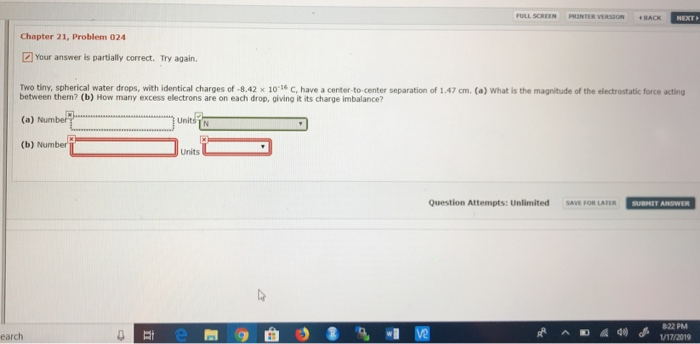FULL SCREEN PRINTER VERSION ·BACK Chapter 21, Problem 024 Your answer is partially correct. Try again. Two tiny, spherical water drops, with identical charges of -8.42 x 10 16 C, have a center-to-center separation of 1.47 cm. (a) What is the magnitude of the electrostatic force acting between them? (b) How many excess electrons are on each drop, giving it its charge imbalance? (b) Number Units Question Attempts: Unlimited SAVE FOR LATER SUBMET ANSWER earch